A prime number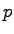is called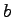-elite if only finitely many generalized Fermat numbers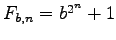are quadratic residues modulo. Letbe a prime. Write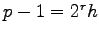with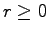and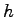odd. Define the length of the b-Fermat period ofto be the minimal natural number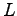such that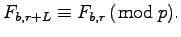Recently Müller and Reinhart derived three conjectures on-elite primes, two of them being the following. (1) For every natural number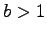there is a-elite prime. (2) There are generalized elite primes with elite periods of arbitrarily large lengths. We extend Müller and Reinhart's observations and computational results to further support above two conjectures. We show that Conjecture 1 is true for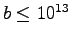and that for every possible length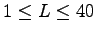there actually exists a generalized elite prime with elite period length.